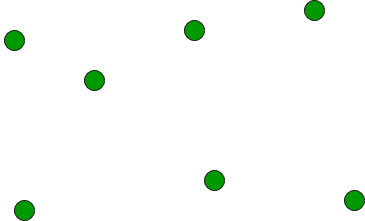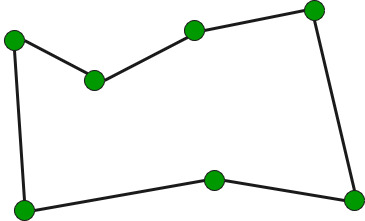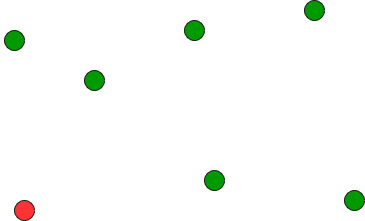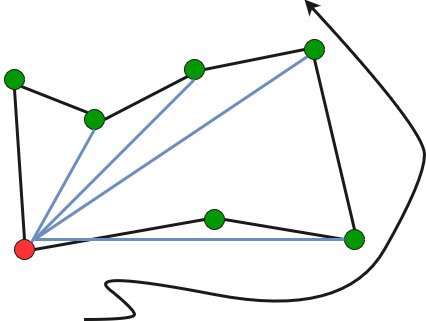# Find Simple Closed Path for a given set of points

• Difficulty Level : Hard
• Last Updated : 13 Jul, 2022

Given a set of points, connect the dots without crossing.Example:

```Input: points[] = {(0, 3), (1, 1), (2, 2), (4, 4),
(0, 0), (1, 2), (3, 1}, {3, 3}};

Output: Connecting points in following order would
not cause any crossing
{(0, 0), (3, 1), (1, 1), (2, 2), (3, 3),
(4, 4), (1, 2), (0, 3)}```

We strongly recommend you to minimize your browser and try this yourself first.
The idea is to use sorting.

• Find the bottom-most point by comparing y coordinate of all points. If there are two points with same y value, then the point with smaller x coordinate value is considered. Put the bottom-most point at first position.• Consider the remaining n-1 points and sort them by polor angle in counterclockwise order around points. If polor angle of two points is same, then put the nearest point first.
• Traversing the sorted array (sorted in increasing order of angle) yields simple closed path.How to compute angles?
One solution is to use trigonometric functions.
Observation: We don’t care about the actual values of the angles. We just want to sort by angle.
Idea: Use the orientation to compare angles without actually computing them!

Below is C++ implementation of above idea.

## C++

 `// A C++ program to find simple closed path for n points` `// for explanation of orientation()` `#include ` `using` `namespace` `std;`   `struct` `Point` `{` `    ``int` `x, y;` `};`   `// A global point needed for  sorting points with reference` `// to the first point. Used in compare function of qsort()` `Point p0;`   `// A utility function to swap two points` `int` `swap(Point &p1, Point &p2)` `{` `    ``Point temp = p1;` `    ``p1 = p2;` `    ``p2 = temp;` `}`   `// A utility function to return square of distance between` `// p1 and p2` `int` `dist(Point p1, Point p2)` `{` `    ``return` `(p1.x - p2.x)*(p1.x - p2.x) +` `           ``(p1.y - p2.y)*(p1.y - p2.y);` `}`   `// To find orientation of ordered triplet (p, q, r).` `// The function returns following values` `// 0 --> p, q and r are collinear` `// 1 --> Clockwise` `// 2 --> Counterclockwise` `int` `orientation(Point p, Point q, Point r)` `{` `    ``int` `val = (q.y - p.y) * (r.x - q.x) -` `              ``(q.x - p.x) * (r.y - q.y);`   `    ``if` `(val == 0) ``return` `0;  ``// collinear` `    ``return` `(val > 0)? 1: 2; ``// clockwise or counterclock wise` `}`   `// A function used by library function qsort() to sort` `//  an array of points with respect to the first point` `int` `compare(``const` `void` `*vp1, ``const` `void` `*vp2)` `{` `   ``Point *p1 = (Point *)vp1;` `   ``Point *p2 = (Point *)vp2;`   `   ``// Find orientation` `   ``int` `o = orientation(p0, *p1, *p2);` `   ``if` `(o == 0)` `     ``return` `(dist(p0, *p2) >= dist(p0, *p1))? -1 : 1;`   `   ``return` `(o == 2)? -1: 1;` `}`   `// Prints simple closed path for a set of n points.` `void` `printClosedPath(Point points[], ``int` `n)` `{` `   ``// Find the bottommost point` `   ``int` `ymin = points.y, min = 0;` `   ``for` `(``int` `i = 1; i < n; i++)` `   ``{` `     ``int` `y = points[i].y;`   `     ``// Pick the bottom-most. In case of tie, chose the` `     ``// left most point` `     ``if` `((y < ymin) || (ymin == y &&` `         ``points[i].x < points[min].x))` `        ``ymin = points[i].y, min = i;` `   ``}`   `   ``// Place the bottom-most point at first position` `   ``swap(points, points[min]);`   `   ``// Sort n-1 points with respect to the first point.` `   ``// A point p1 comes before p2 in sorted output if p2` `   ``// has larger polar angle (in counterclockwise` `   ``// direction) than p1` `   ``p0 = points;` `   ``qsort``(&points, n-1, ``sizeof``(Point), compare);`   `   ``// Now stack has the output points, print contents` `   ``// of stack` `   ``for` `(``int` `i=0; i

Output:

```(0, 0), (3, 1), (1, 1), (2, 2), (3, 3),
(4, 4), (1, 2), (0, 3), ```

Time complexity of above solution is O(n Log n) if we use a O(nLogn) sorting algorithm for sorting points.
Auxiliary Space: O(1), since no extra space has been taken.

Source:
http://www.dcs.gla.ac.uk/~pat/52233/slides/Geometry1x1.pdf